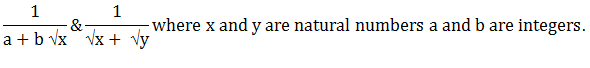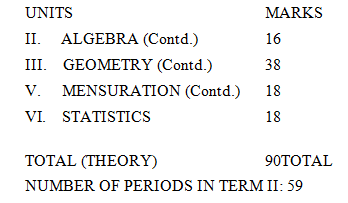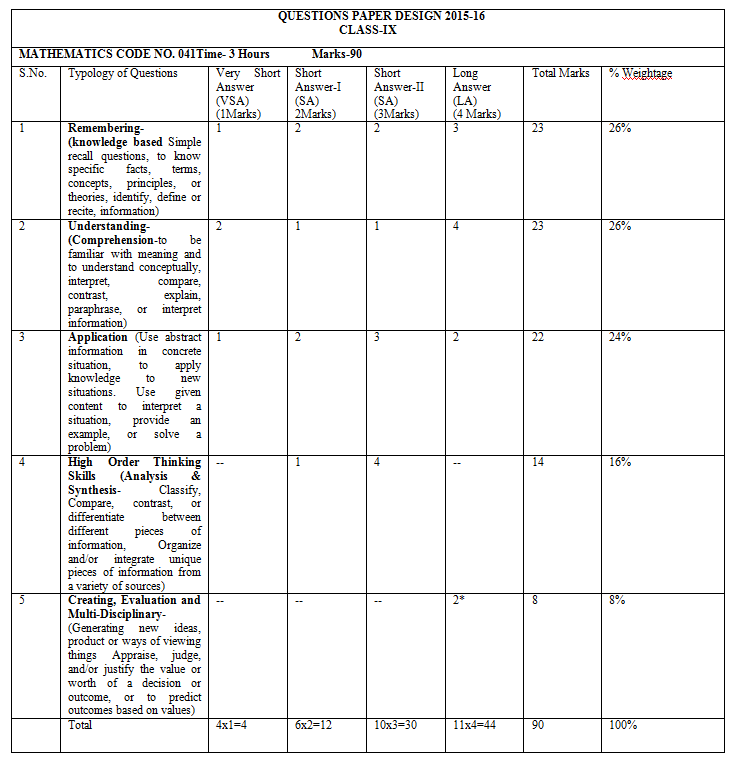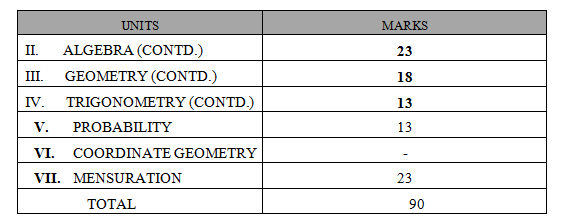Home » 10th Class » Syllabus, Course Structure and Question Paper Design of Mathematics Code No. 041 for Class 9 and 10 under NSQF

# Syllabus, Course Structure and Question Paper Design of Mathematics Code No. 041 for Class 9 and 10 under NSQF

Mathematics

(Code No.041)

The Syllabus in the subject of Mathematics has undergone changes from time to time in accordance with growth of the subject and emerging needs of the society. The present revised syllabus has been designed in accordance with National Curriculum Framework 2005 and as per guidelines given in Focus Group on Teaching of Mathematics which is to meet the emerging needs of all categories of students. Motivating the topics from real life problems and other subject areas, greater emphasis has been laid on applications of various concepts.

The curriculum at Secondary stage primarily aims at enhancing the capacity of students to employ Mathematics in solving day-to-day life problems and studying the subject as a separate discipline. It is expected that students should acquire the ability to solve problems using algebraic methods and apply the knowledge of simple trigonometry to solve problems of height and distances. Carrying out experiments with numbers and tonus of geometry, framing hypothesis and verifying these with further observations form inherent pan of Mathematics learning at this stage. The proposed curriculum includes the study of number system, algebra, geometry, trigonometry, mensuration, statistics, and graphs and coordinates geometry etc.

The teaching of Mathematics should be imparted through activities which may involve the use of concrete materials, models, patterns, charts, pictures, posters, games, puzzles and experiments.

OBJECTIVES

The broad objectives of teaching of Mathematics at secondary stage are to help the learners to:

• consolidate the Mathematical knowledge and skills acquired at the upper primary stage;
• acquire knowledge and understanding, particularly by way of motivation and visualization of basic concepts, terms, principles and symbols and underlying processes and skills;
• develop mastery of basic algebraic skills;
• develop drawing skills;
• feel the flow of reasoning while proving a result or solving a problem;
• apply the knowledge and skills acquired to solve problems and wherever possible by more than one method;
• to develop positive ability to think, analyze and articulate logically;
• to develop awareness of the need for national integration, protection of environment, observance of small family norms, removal of social barriers, elimination of gender biases:
• to develop necessary skills to work with modern technological devices such as calculators, computers etc.
• to develop interest in mathematics as a problem-solving tool in various fields for its beautiful structures and patterns ;
• to develop reverence and respect towards great Mathematicians for their contributions to the field of Mathematics;
• to develop interest in the subject by participating in related competitions;
• to acquaint students with different aspects of mathematics used in daily life;
• to develop an interest in students to study mathematics as a discipline.

General Instructions:

• As per CCE guidelines, the syllabus of Mathematics for classes IX and X has been divided term wise.
• The units specified for each term shall be assessed through both formative and summative assessments.
• In each term, there will be two formative assessments, each earning 10% weightage and out of the four FA one would be problem solving assessment (PSA).
• The summative assessment in term I will carry 30% weightage and the summative assessment in the term II will also carry 30% weightage.
• Listed laboratory activities and projects will necessarily be assessed through formative assessments.

Course Structure

Class-IX

First Term                                                                                                                 Marks: 90

UNITS                                                                                                                         MARKS

1.) NUMBER SYSTEMS                                                                                                        17

2.)ALGEBRA                                                                                                                          25

3.)GEOMETRY                                                                                                                      37

4.)COORDINATE GEOMETRY                                                                                         06

5.)MENSURATION                                                                                                              05

TOTAL (THEORY)                                                                                                                  90

TOTAL NUMBER OF PERIODS IN TERM I: 77

UNIT I: NUMBER SYSTEMS                           (16 Periods)

NUMBER SYSTEMS

1. Review of representation of natural numbers, integers, and rational numbers on the number line. Rational numbers as recurring/terminating decimals.
2. Examples of non-recurring/ non terminating decimals such as Ö2, Ö3, Ö5 etc. Existence of non-rational numbers (irrational numbers) such as Ö2, Ö3 and their representation on the number line.Rational numbers as recurring/terminating decimals.
3. Definition of nth root of a real number.
4. Recall of laws of exponents with integral powers. Rational exponents with positive real bases (to be done by particular cases, allowing learner to arrive at the general laws.)
5. Rationalization (with precise meaning) of real numbers of the type & their combinationsUNIT II: ALGEBRA                                                                                 (23) Periods

1. POLYNOMIALS

Definition of a polynomial in one variable, its coefficients, with examples and counter examples, its terms, zero polynomial. Degree of a polynomial. Constant, linear, quadratic and cubic polynomials; monomials, binomials, trinomials. Factors and multiples. Zeros of a polynomial. State and motivate the Remainder Theorem with examples(and analogy to integers). Statement and proof of the Factor Theorem. Factorization of ax2 + bx + c, a ≠ 0 where a, b and c are real numbers, and of cubic polynomials using the Factor Theorem.

Recall of algebraic expressions and identities. Further verification of identities of the type (x + y + z)2 =x2 + y2 + z2+ 2xy + 2yz + 2zx, (x ± y)3 = x3 ± y3 ± 3xy(x ± y), x3± y3 = (x ± y) (x2 ± xy + y2),x3 + y3+ z3 — 3xyz = (x + y + z) (x2 + y2 + z2 — xy — yz — zx) and their use in factorization of polynomials. Simple expressions reducible to these polynomials.

UNIT III:GEOMETRY                                                                           (27) Periods

1. LINES AND ANGLES
• (Motivate) If a ray stands on a line, then the sum of the two adjacent angles so formed is 1800and the converse.
1. (Prove) If two lines intersect, the vertically opposite angles are equal.
2. (Motivate) Results on corresponding angles, alternate angles, interior angles when a transversal intersects two parallel lines.
3. (Motivate) Lines, which are parallel to a given line, are parallel.
4. (Prove) The sum of the angles of a triangle is 1800.
5. (Motivate) If a side of a triangle is produced, the exterior angle so formed is equal to the sum of the two interior opposite angles.
6. TRIANGLES
7. (Motivate) Two triangles are congruent if any two sides and the included angle of one triangle is equal to any two sides and the included angle of the other triangle (SAS Congruence).
8. (Prove) Two triangles are congruent if any two angles and the included side of one triangle is equal to any two angles and the included side of the other triangle (ASA Congruence).
9. (Motivate) Two triangles are congruent if the three sides of one triangle are equal to three sides of the other triangle (SSS Congruence).
10. (Motivate) Two right triangles are congruent if the hypotenuse and a side of one triangle are equal (respectively) to the hypotenuse and a side of the other triangle.
11. (Prove) The angles opposite to equal sides of a triangle are equal.
12. (Motivate) The sides opposite to equal angles of a triangle are equal.

UNIT IV: COORDINATE GEOMETRY                                      (09) Periods

COORDINATE GEOMETRY

The Cartesian plane coordinates of a point, names and terms associated with the coordinate plane, notations, plotting points in the plane

UNIT V:MENSURATION                                                               (02) Periods

AREAS

Introduction, Area of a Triangle using Heron’s Formula (without proof)

Course Structure

Class-IX

Second Term                                                                                       Marks: 90**Note: The text of OTBA for SA-II will be from Unit-II: Linear Equations in two variables

UNIT II: ALGEBRA (Contd.)                                                              (10) Periods

2.) LINEAR EQUATIONS IN TWO VARIABLES

Recall of linear equations in one variable. Introduction to the equation in two variables. Prove that a linear equation in two variables has infinitely many solutions and justify their beings written as ordered pairs of real numbers, plotting them and showing that they seem to lie on a line. Examples, problems from real life, including problems on Ratio and Proportion and with algebraic and graphical solutions being done simultaneously

UNIT III: GEOMETRY (Contd.)                                               (26) Periods

All Properties of quadrilateral and theorems to be introduced by exploratory approach

1. The diagonal divides a parallelogram into two congruent triangles
2. In a parallelogram opposite sides are equal, and conversely
3. In a parallelogram opposite angles are equal and conversely
4. A quadrilateral is a parallelogram if a pair of its opposite sides is parallel and equal
5. In a parallelogram, the diagonals bisect each other and conversely
6. In a triangle, the line segment joining the mid points of any two sides is parallel to the third side and its converse.

4.) AREA

Review concept of area, recall area of a rectangle.

1. (Prove) Parallelograms on the same base and between the same parallels have the same area.
2. (Motivate) Triangles on the same base and between the same parallels are equal in area.

5.) CIRCLES

Through examples, arrive at definitions of circle related concepts, radius, circumference, diameter, chord, arc, subtended angle.

1. (Prove) Equal chords of a circle subtend equal angles at the center and (motivate) its converse.
1. (Motivate) The perpendicular from the center of a circle to a chord bisects the chord and conversely, the line drawn through the center of a circle to bisect a chord is perpendicular to the chord.
2. (Motivate) There is one and only one circle passing through three given non-collinear points.
3. (Motivate) Equal chords of a circle (or of congruent circles) are equidistant from the centre(s) and conversely.
4. (Prove) The angle subtended by an arc at the center is double the angle subtended by it at any point on the remaining part of the circle.
5. (Motivate) Angles in the same segment of a circle are equal.
6. (Motivate) If a line segment joining two points subtends equal angle at two other points lying on the same side of the line containing the segment, the four points lie on a circle.
7. (Motivate) The sum of the either pair of the opposite angles of a cyclic quadrilateral is 1800 and its converse.

UNIT V: MENSURATION (Contd.)                                                  (10) Periods

SURFACE AREAS AND VOLUMES

Surface areas and volumes of cubes, cuboids &spheres (including hemispheres) and right circular cones/ cylinder

UNIT VI: STATISTICS                                                                             (13) Periods

STATISTICS

Introduction to Statistics: Collection of data, presentation of data — tabular form, ungrouped / grouped, bar graphs, histograms (with varying base lengths), frequency polygons.Mean, median, mode of ungrouped data.

Question Paper Design Class 9Note:  The question paper will include a section of open text based assessment (question of 10 marks).  The case study will be supplied to students in advance. The case studies are designed to text the analytical and higher order thinking skills of students.

*Out of the LA (4 marks) will be to assess the values inherent in the texts.

MATHS

CLASS – X

TERM 1                                                                          MARKS: 90TOTAL NUMBER OF PERIODS IN TERM I: 80

UNIT I: NUMBER SYSTEMS

UNIT II: Algebra                                                                                                              (22) Periods

1. Polynomials:

Zeros of a polynomial. Relationship between zeros and coefficients of quadratic polynomials. Statement and simple problems on division algorithm for polynomials with real coefficients.

1. Pair of Linear Equations in two Variables:

Pair of linear equations in two variables and their graphical solution. Geometric representation of different possibilities of solutions/inconsistency.

Algebraic conditions for number of solutions. Solution of a pair of linear equations in two variables algebraically – by substitution, by elimination and by cross multiplication method. Simple situational problems must be included. Simple problems on equations reducible to linear equations may be included.

UNIT III: GEOMETRY                                                                (15) Periods

1. TRIANGLES

Definitions, examples, counterexamples of similar triangles.

1. (Prove) If a line is drawn parallel to one side of a triangle to intersect the other two sides in distinct points, the other two sides are divided in the same ratio.
2. (Motivate) If a line divides two sides of a triangle in the same ratio, the line is parallel to the third side.
3. (Motivate) If in two triangles, the corresponding angles are equal, their corresponding sides are proportional and the triangles are similar.
4. (Motivate) If the corresponding sides of two triangles are proportional, their corresponding angles are equal and the two triangles are similar.
5. (Motivate) If one angle of a triangle is equal to one angle of another triangle and the sides including these angles are proportional, the two triangles are similar.
6. (Motivate) If a perpendicular is drawn from the vertex of the right angle of a light triangle to the hypotenuse, the triangles on each side of the perpendicular are similar to the whole triangle and to each other.
7. (Prove) The ratio of the areas of two similar triangles is equal to the ratio of the squares on their corresponding sides.
8. (Prove) In a right triangle, the square on the hypotenuse is equal to the sum of the squares on the other two sides.
9. (Prove) In a triangle, if the square on one side is equal to sum of the squares on the other two sides, the angles opposite to the first side is a right triangle.

UNIT IV: TRIGONOMETRY                                                          (25) Periods

1. INTRODUCTION TO TRIGONOMETRY

Trigonometric ratios of an acute angle of a right-angled triangle. Proof of their existence (well defined); motivate the ratios, whichever are defined at 0o& 90o. Values (with proofs) of the trigonometric ratios of 30o, ,  45o&60o.Relationships between the ratios.

1. TRIGONOMETRIC IDENTITIES

Proof and applications of the identity sin2A + cos2A = 1. Only simple identities to be given. Trigonometric ratios of complementary angles.

UNIT V: STATISTICS                                                                      (18) Periods

1. STATISTICS

Mean median and mode of grouped data (bi-modal situation to be avoided). Cumulative frequency graph.

MATHS

CLASS – X

TERM II                                                                      MARKS: 90TOTAL NUMBER OF PERIODS IN TERM II: 55

Unit II: Algebra(Contd.)                                                                      (15) periods

Standard form of a quadratic equation ax2+ bx + c = 0, (a ≠ 0). Solution of the quadratic equations (only real roots) by factorization, by completing the square and by using quadratic formula. Relationship between discriminant and nature of roots. Problems related to day to day activities to be incorporated.

4.) ARITHMETIC PROGRESSION

Motivation for studying Arithmetic progression, Derivation of Standard results of finding the nth term and sum of first n terms and their application in solving daily life problems.

UNIT III: GEOMETRY (Contd.)                                                     (8) Periods

5.) CIRCLES

Tangents to a circle at a point.

1. (Prove) The tangent at any point of a circle is perpendicular to the radius through the point of contact.
2. (Prove) The lengths of tangents drawn from an external point to circle are equal.

UNIT IV: TRIGONOMETRY                                                    (6) Periods

6.) HEIGHTS AND DISTANCES

Simple and believable problems on heights and distances.  Problems should not involve more than two right triangles.  Angle of elevation/depression should be only 30°, 45°, 60°.

UNIT V: STATISTICS AND PROBABILITY                                   (6) periods

PROBABILITY

Classical definition of probability, simple problems on single events (not using set notation)

UNIT VII: MENSURATION                                                             (20) Periods

1.) AREAS RELATED TO CIRCLES

Motivate the area of a circle; area of sectors and segments of a circle. Problems based on areas and perimeter / circumference of the above said plane figures. (In calculating area of segment of a circle, problems should be restricted to central angle of 60°, 90″ & 120″ only. Plane figures involving triangles, simple quadrilaterals and circle should be taken.)

2.) SURFACE AREAS AND VOLUMES

• Problems on finding surface areas and volumes of combinations of any two of the following; cubes, cuboids, spheres, hemispheres and right circular cylinders/cones. Frustum of a cone.
• Problems involving converting one type of metallic solid into another and other mixed problems. (Problems with combination of not more than two different solids be taken.

Question Paper Design Class 10

RECOMMENDED BOOKS

1. Mathematics- Textbook for class IX – NCERT Publication
2. Mathematics – Textbook for class X – NCERT Publication
3. Guidelines for Mathematics laboratory in schools, class IX – CBSE Publication
4. Guidelines for Mathematics laboratory in schools, class X-CBSE Publication
5. A hand book for designing mathematics laboratory in schools- NCERT Publication
6. Laboratory manual – Mathematics, secondary stage – NCERT Publication.
7. Mathematics exemplar problems for class IX, NCERT publication
8. Mathematics Exemplar problems for class X, NCERT publicationI am a person who is positive about every aspect of life.I am a Computer Science lecturer in a school. I love to spend my free time in learning new things.Working for eVirtualGuru gives me a lot extra knowledge and immense joy.